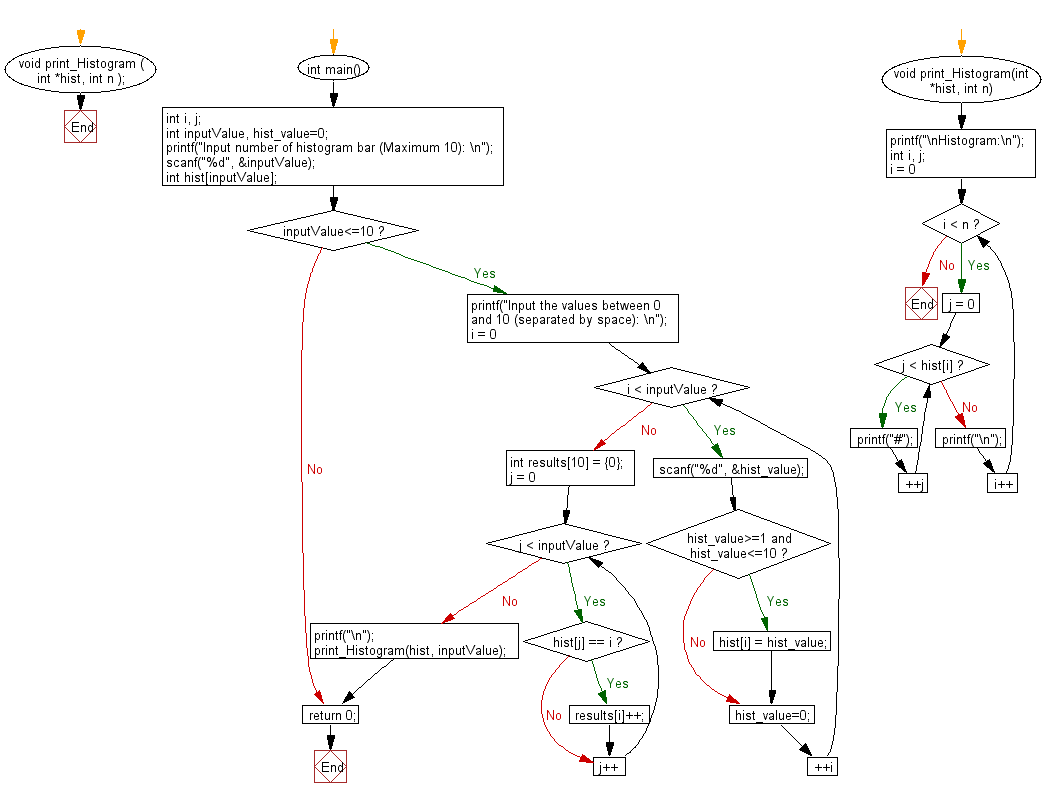﻿ C Program: Print a histogram based on some integer values

# C Exercises: Takes some integer values from the user and print a histogram.

## C Basic Declarations and Expressions: Exercise-99 with Solution

Write a C program that takes some integer values from the user and prints a histogram.

Sample Solution:

C Code:

``````#include <stdio.h>

// Function to print a histogram
void print_Histogram ( int *hist, int n );

int main() {
int i, j;
int inputValue, hist_value=0;

// Prompt the user to input the number of histogram bars (Maximum 10)
printf("Input number of histogram bar (Maximum 10): \n");
scanf("%d", &inputValue);
int hist[inputValue];
if (inputValue<=10)
{
// Prompt the user to input values for the histogram bars
printf("Input the values between 0 and 10 (separated by space): \n");
for (i = 0; i < inputValue; ++i) {
scanf("%d", &hist_value);
// Check if the input value is within the valid range (1 to 10)
if (hist_value>=1 && hist_value<=10)
hist[i] = hist_value;
hist_value=0;
}

int results = {0};
// Count the occurrences of each value in the histogram
for(j = 0; j < inputValue; j++) {
if ( hist[j] == i){
results[i]++;
}
}

printf("\n");
// Print the histogram
print_Histogram(hist, inputValue);
}
return 0;
}

// Function to print the histogram
void print_Histogram(int *hist, int n) {
printf("\nHistogram:\n");
int i, j;
// Loop through the histogram bars and print '#' for each occurrence
for (i = 0; i < n; i++) {
for ( j = 0; j < hist[i]; ++j) {
printf("#");
}
printf("\n");
}
}

``````

Sample Output:

```Input number of histogram bar (Maximum 10):
4
Input the values between 0 and 10 (separated by space):
9
7
4
3

Histogram:
#########
#######
####
###

```

Flowchart:C programming Code Editor:

What is the difficulty level of this exercise?

Test your Programming skills with w3resource's quiz.

﻿